# SpinalHDL(八):开发仿真测试一条龙

SpinalHDL就是这样的汉子，一次又一次的轻视它，但它又不时的给你惊喜。

• 不同于iotest的peak poke， 它的后台是verilator, verilator非常强大，性能比起商业软件VCS、NC毫不逊色。
• spinal.sim 通过包装verilator提供的VPI，能够很方便灵活，实时的跟dut交互通信, 加上Scala本身语言的灵活性，它能做的不比UVM少。

### 一：简单的数字混频器

class FreqMixer(cfg: FreqMixerConfig) extends Component{
val io = new Bundle{
....
}
....
}

class FreqMixerTB(cfg: FreqMixerConfig) extends FreqMixer(cfg){//简单继承即可
//这样FreqMixer内的接口和变量完全可见
def init(){
clockDomain.forkStimulus(5) //clockDomain可见
io.din.valid #= false       //io 可见
}
def forcePara(thetaStep： Long, thetaInit: Long){
io.thetaStep  #= thetaStep  //配置相位步长
io.thetaInit  #= thetaInit  //配置相位初始值
}
def forkData(source) ....
def forkCompare().....
}


dut.forcePara((2*Pi/40).fixAsLong(SQ(32,28), 0)//设置2Pi/40的,相当于一个正玄波周期40个样点
dut.forkData(List.fill(1000)(0.5+0*j).fixAsLong(SQ(8,7)))  //灌入直流，SQ(8,7)量化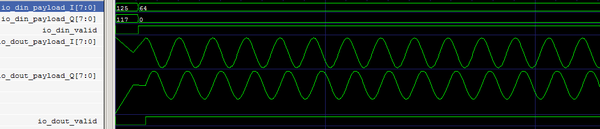val source = List.fill(1000)(0.5+0*j)
val sourceWithNoise = AWGN(source, snr = 3) //添加一组信噪比为3dB的高斯白噪声
dut.forkData(soureWithNoise.fixAsLong)
dut.forcePara((2*Pi/200).fixAsLong(SQ(32,28), 0)//同时把周期设大一点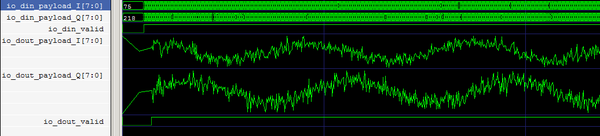val sourceWithNoise = AWGN(source, snr = -3) //信噪比设为 -3dB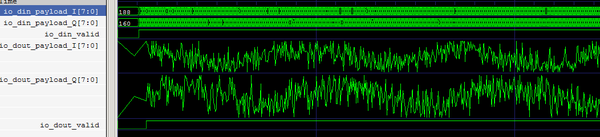val sourceWithNoise = GainTo(AWGN(source, snr = -3), -9) //信噪比-3dB，功率增益调到 -9dB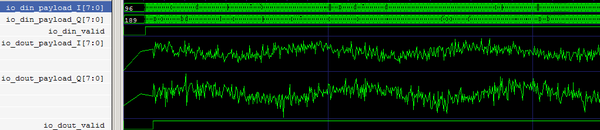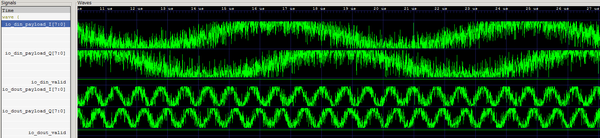#### 添加总线读写函数

class GPSTop extends Component{
val io = new Bundle{
...
val ahb = AHBLite3(AHBLite3Config(32,32))
}
}


implicit class AhbLite3Extends(ahb: AhbLite3){ //给AHBlite3隐式扩展simWrite, simReda方法
import spinal.core._
import spinal.core.sim._

def simWrite(addr: Long, data: BigInt)(cd: ClockDomain) = {
cd.waitSampling()// 等待时钟沿
ahb.HSEL   #= true
ahb.HWRITE #= true
ahb.HTRANS #= 2
cd.waitSampling()// 等待时钟沿
ahb.HWDATA #= data
}

cd.waitSampling() // 等待时钟沿
ahb.HSEL   #= true
ahb.HWRITE #= false
ahb.HTRANS #= 2
cd.waitSampling()// 等待时钟沿
sleep(0)
ahb.HRDATA.toLong
}
}


class GPSTopTB extends GPSTOP{

def forceGPSPara(cs: GPSCase) = { //可以进一步封装寄存器配置函数
write(0x00,  cs.para.modes)
write(0x04,  cs.para.parameter0)
write(0x08,  cs.para.parameter0)
write(0x0c,  cs.para.intEnables)
.......
write(0x40,  cs.para.....)
}
}


### 二：验证CASE规划测试回归

• 一类是交互类，异常的测试，
• 还有一类是算法功能测试，由算法来生成向量集

//GPS发送case， 卫星编号， 信噪比，位置偏移， 频偏一目了然， 实现细节全部封装在对应的类里面
object GPSTxCases {
val case00: List[SatCase] = List(
SatCase(Sat(GPS,  1), ChannelCase(snr = 100, chipOffset = 0, Fdoppler = 0 Hz)))

....
val case04 : List[SatCase] = List(
SatCase(Sat(GPS,  1 ), ChannelCase(snr =  0, chipOffset = 399 , Fdoppler = 10   Hz)),
SatCase(Sat(GPS,  31), ChannelCase(snr =  0, chipOffset = 100 , Fdoppler = 500 kHz)),
SatCase(Sat(GPS,  7),  ChannelCase(snr =  0, chipOffset = 100 , Fdoppler = 500 kHz)),
SatCase(Sat(GPS,  15), ChannelCase(snr = -2, chipOffset = 2047, Fdoppler = 27   Hz)))
...
}


object GPSTECases {
val case00 = TECase(GPS,
sendcase = GPSTxCases.case00,
jobs     = GPSTEJobs.case00,
maxMs    = 32
)

val case01 = TECase(GPS,
sendcase = GPSTxCases.case01,
jobs     = GPSTEJobs.case01,
maxMs    = 32
)


class TESimRegression extends  FunSuite {
test("tesim_case00"){
val s = new TESim(GnssConfig(),
GPSTECases.case00,//force GPS信号以及跟踪job
BDTECases.case00, //force BD信号以及跟踪job
withWave = false,
dumpNode = false)
s.sim(ms = 30)
}

...

test("tesim_caseN"){
...
}
}


### 三：开源工具

verilator 相比VCS速度上几乎没区别，唯一的gtkWave相比verdi要弱一些，我自己本来是一个重度的Verid依赖着，以为离开verdi 都不会看波形，经过一段时间的适应gtkWave好像也没那么糟糕，配合上IDEA的追踪，并且SpinalHDL生成的代码会原封不动的保留信号名，所以快速定位追踪波形没有任何问题。当你遍历回归时，可以关闭文件dump，以及波形，速度会更快。